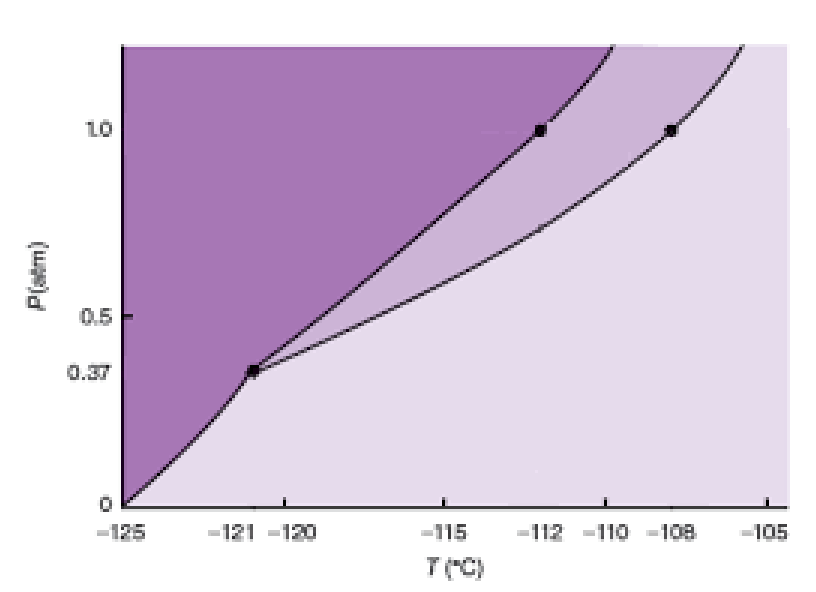# Problem: The phase diagram for xenon is shown below:If the pressure exerted on a xenon sample is 0.75 atm, and the temperature is -114°C, in what phase does xenon exist?

###### FREE Expert Solution

Analyze the given phase diagram and determine the phase of Xe at -114°C and 0.75 atm

Recall that a phase diagram shows the transition of matter between solid, liquid, and gas phases as temperature and pressure changes.

In a phase diagram, the different phases can be identified by their location:

• Solid: can be found at high pressure and low temperature

• Liquid: can be found between the solid and gas regions

• Gas: can be found at low pressure and high temperature

93% (380 ratings)###### Problem Details

The phase diagram for xenon is shown below:If the pressure exerted on a xenon sample is 0.75 atm, and the temperature is -114°C, in what phase does xenon exist?

Frequently Asked Questions

What scientific concept do you need to know in order to solve this problem?

Our tutors have indicated that to solve this problem you will need to apply the Phase Diagram concept. You can view video lessons to learn Phase Diagram. Or if you need more Phase Diagram practice, you can also practice Phase Diagram practice problems.

What is the difficulty of this problem?

Our tutors rated the difficulty ofThe phase diagram for xenon is shown below:If the pressure e...as low difficulty.